Definitions of Square Dance Calls and Concepts

Index -->  Plus  |  A1  |  A2  |  C1  |  C2  |  C3A  |  C3B  |  C4  |  NOL  |
Definitions (Text Only) -->  Plus  |  A1  |  A2  |  C1  |  C2  |  C3A  |  C3B  |  C4  |  NOL  |
 Find call:

\$BE,@Z\$J(B 2 x 4 \$B\$+\$i(B.

Ends \$B\$O(B Split Circulate \$B\$r\$7(B, Centers \$B\$O(B Split Counter Rotate 1/4 \$B\$r\$7\$^\$9(B.

2 x 4 \$B\$G=*\$o\$j\$^\$9(B.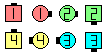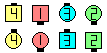Lickety Split\$B\$NA0(B \$B8e(B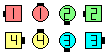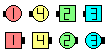Lickety Split\$B\$NA0(B \$B8e(B

\$BCm
• \$BA4BN\$N(B formation \$B\$G(B End \$B\$H(B Center \$B\$N\$I\$A\$i\$K\$\$\$k\$+\$r8+\$F\$+\$i(B, \$B%3!<%k\$N<+J,\$N%Q!<%H\$r(B Split \$B\$G9T\$\$\$^\$9(B.
• \$BNr;KE*\$K(B, \$B\$"\$k%3!<%i!<\$O(B Diagonal Box, Jay, Once Removed, Trapezoid \$B\$N\$h\$&\$J%3%s%;%W%H\$H0l=o\$K\$3\$N%3!<%k\$r;H\$\$\$^\$7\$?(B. \$BH`\$i\$O(B, \$BA4BN\$N(B 8 \$B?M\$N(B formation \$B\$G(B End \$B\$+(B Center \$B\$+\$r8+\$F(B, \$B;X<(\$5\$l\$?(B formation \$B\$G%3!<%k\$N<+J,\$N%Q!<%H\$r\$9\$k\$h\$&\$K\$7\$F\$\$\$^\$7\$?(B. \$B\$3\$l\$O(B, \$B:#\$O\$R\$s\$7\$e\$/\$rGc\$&;H\$\$J}\$G\$9(B.
• Lickety Split \$B\$O%3!<%i!<\$K\$H\$C\$F;H\$\$0W\$\$%3!<%k\$G\$9(B. \$BIaDL\$N(B formation \$B\$+\$i(B T-Bone formation \$B\$K\$7\$?\$j(B, \$B5U\$K(B T-Bone \$B\$+\$iIaDL\$KLa\$9\$3\$H\$,\$G\$-\$k\$+\$i\$G\$9(B.
• \$B1~MQ(B: Magic (Column) Lickety Split.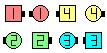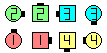Magic Lickety Split\$B\$NA0(B Ends Magic Split Circulate asCenters Magic Split Counter Rotate 1/4\$B\$N8e(B (\$B=*\$o\$j(B)Choreography for Lickety SplitComments? Questions? Suggestions?

https://www.ceder.net/def/licketysplit.php?level=C3B&language=japan
22-September-2021 04:41:16